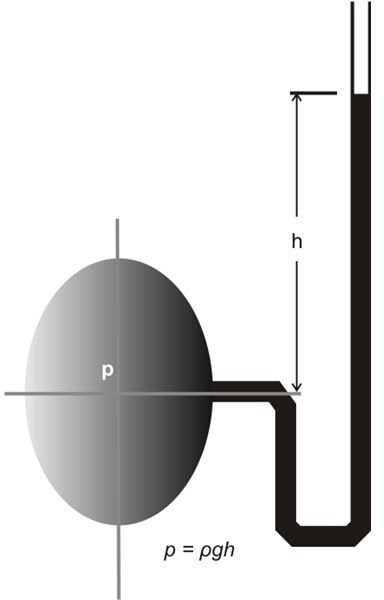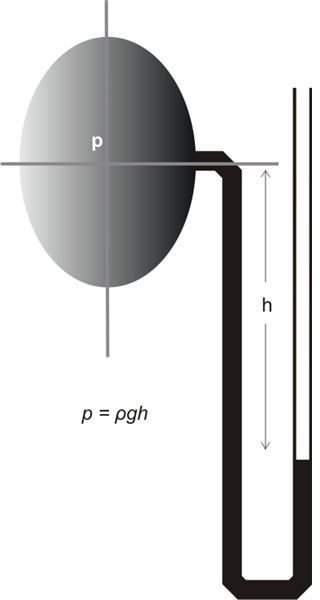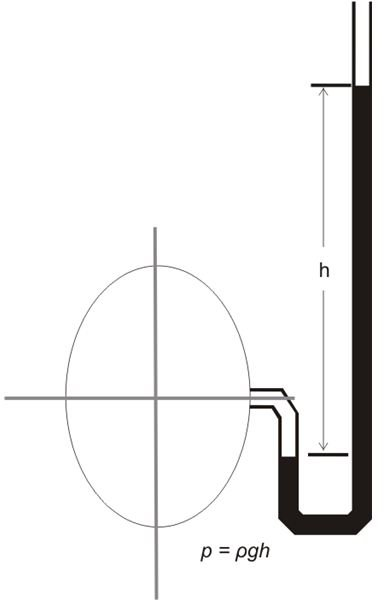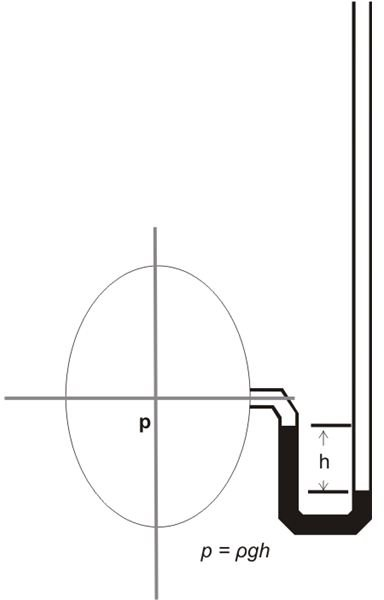# How a U Tube Manometer Works

## How to Use a U-Tube Manometer

We learnt and assessed the hydrostatic law through one of my previous articles and derived the following law of pressure:

dp/dz = ρg

Basically the method of measuring fluid pressures through the above hydrostatic law is known as manometry and the employed device is called a manometer.

Although there may be various types of manometers, here we discuss one of the more efficient one, used extensively for the purpose – the U tube manometer.

As the name refers to, a U-tube manometer is made up of two parallel tubes joined together in the form of the letter “U.”

The two parallel tubes basically constitute the limbs of the device.

Whether it’s for the measurement of pressure in a fluid, liquid, or gas, U-tube manometers may be employed for most purposes. They may be used in different positions such as inverted, upright, or inclined.

The following examples will walk us through a few typical applications of this important device and the methods employed in the measurement of fluid and gas pressures under different situations, let’s examine them:

Liquid under Average Pressure: In this case, a U-tube manometer is simply attached as shown in the diagram below. The liquid level rises by a height h in the tube with the open end, over the point where the pressure is being measured. The height h is monitored and calculated such that the pressure exerted at the point equals the pressure exerted by the column h.

p = ρghLiquid Pressure under Vacuum: In such conditions, a U-tube manometer is connected primarily under the point where the vacuum is to be measured. This enables the depression h of the liquid level in the tube having the open end such that the vacuum at the measured point equals the depression h of the fluid due to the vacuum (see figure.)

p = –ρghGas under Pressure: Depending upon the type of gas involved, firstly the liquid enclosed inside the manometer is appropriately selected, so that the acquired measurements (height h) of the fluid inside the tube is well optimized producing wider range of readings over the entire tube. Also the two parameters (gas and the enclosed liquid) are made sure to be completely immiscible by property.

The excess height h of the selected fluid thus obtained in relation to the pressure p of the gas in the open ended tube is given as:

p = ρmgh

Where ρm is the density of the fluid enclosed inside the manometer.

In the above expression, the gas density is not take into account as the hydrostatic pressure of a gas may be negligibly small compared to the density of the fluid and therefore ignored.Gas under Vacuum: As shown in the figure, to measure the pressure of a gas under pressure, the U-tube containing the selected fluid with density ρm is mainly connected at a position under the point where the vacuum is measured. The following expression gives the relation between the pressure p and the resultant depression h in the open ended manometer tube:

p = –ρmgh## Reference

Author: Kumar K.L.

Book: Engineering Fluid Mechanics.

Publisher: S. Chand and Company Limited,

Date: 1992